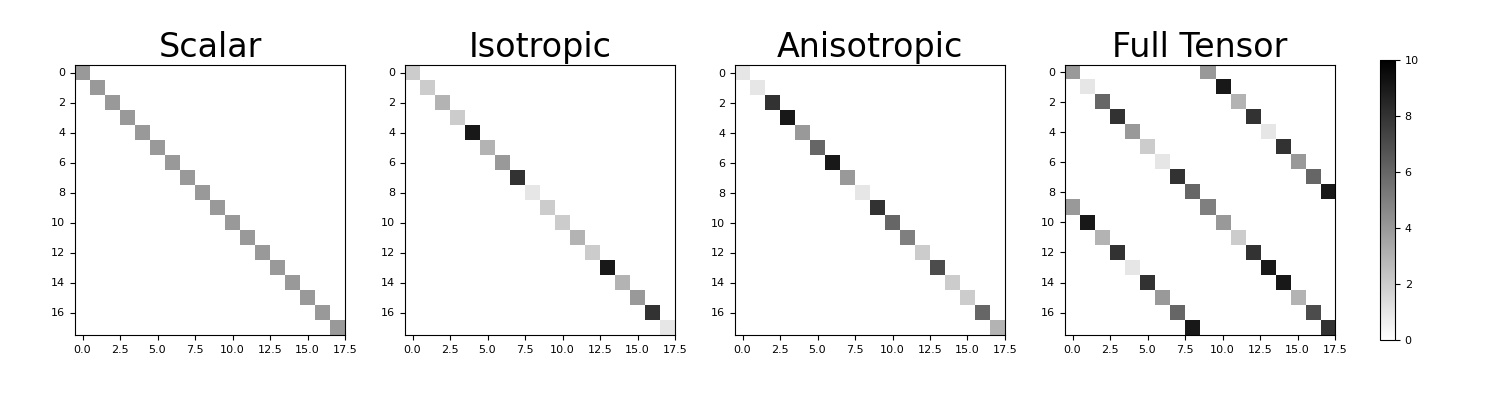# discretize.utils.make_property_tensor¶

discretize.utils.make_property_tensor(mesh, tensor)[source]

Construct the physical property tensor.

For a given mesh, the input parameter tensor is a numpy.ndarray defining the constitutive relationship (e.g. Ohm’s law) between two discrete vector quantities $$\boldsymbol{j}$$ and $$\boldsymbol{e}$$ living at cell centers. The function make_property_tensor constructs the property tensor $$\boldsymbol{M}$$ for the entire mesh such that:

>>> j = M @ e


where the Cartesian components of the discrete vector for are organized according to:

>>> e = np.r_[ex, ey, ez]
>>> j = np.r_[jx, jy, jz]

Parameters
meshdiscretize.base.BaseMesh

A mesh

tensornumpy.ndarray or a float
• Scalar: A float is entered.

• Isotropic: A 1D numpy.ndarray with a property value for every cell.

• Anisotropic: A (nCell, dim) numpy.ndarray where each row defines the diagonal-anisotropic property parameters for each cell. nParam = 2 for 2D meshes and nParam = 3 for 3D meshes.

• Tensor: A (nCell, nParam) numpy.ndarray where each row defines the full anisotropic property parameters for each cell. nParam = 3 for 2D meshes and nParam = 6 for 3D meshes.

Returns
(dim * n_cells, dim * n_cells) scipy.sparse.coo_matrix

The property tensor.

Notes

The relationship between a quantity and its response to external stimuli (e.g. Ohm’s law) in each cell can be defined by a scalar function $$\sigma$$ in the isotropic case, or by a tensor $$\Sigma$$ in the anisotropic case, i.e.:

$\vec{j} = \sigma \vec{e} \;\;\;\;\;\; \textrm{or} \;\;\;\;\;\; \vec{j} = \Sigma \vec{e}$

where

$\begin{split}\Sigma = \begin{bmatrix} \sigma_{xx} & \sigma_{xy} & \sigma_{xz} \\ \sigma_{xy} & \sigma_{yy} & \sigma_{yz} \\ \sigma_{xz} & \sigma_{yz} & \sigma_{zz} \end{bmatrix}\end{split}$

Examples

For the 4 classifications allowable (scalar, isotropic, anistropic and tensor), we construct and compare the property tensor on a small 2D mesh. For this example, note the following:

• The dimensions for all property tensors are the same

• All property tensors, except in the case of full anisotropy are diagonal sparse matrices

• For the scalar case, the non-zero elements are equal

• For the isotropic case, the non-zero elements repreat in order for the x, y (and z) components

• For the anisotropic case (diagonal anisotropy), the non-zero elements do not repeat

• For the tensor caes (full anisotropy), there are off-diagonal components

>>> from discretize.utils import make_property_tensor
>>> from discretize import TensorMesh
>>> import numpy as np
>>> import matplotlib.pyplot as plt
>>> import matplotlib as mpl


Define a 2D tensor mesh

>>> h = [1., 1., 1.]
>>> mesh = TensorMesh([h, h], origin='00')


Define a physical property for all cases (2D)

>>> sigma_scalar = 4.
>>> sigma_isotropic = np.random.randint(1, 10, mesh.nC)
>>> sigma_anisotropic = np.random.randint(1, 10, (mesh.nC, 2))
>>> sigma_tensor = np.random.randint(1, 10, (mesh.nC, 3))


Construct the property tensor in each case

>>> M_scalar = make_property_tensor(mesh, sigma_scalar)
>>> M_isotropic = make_property_tensor(mesh, sigma_isotropic)
>>> M_anisotropic = make_property_tensor(mesh, sigma_anisotropic)
>>> M_tensor = make_property_tensor(mesh, sigma_tensor)


Plot the property tensors.

Expand to show scripting for plot
>>> M_list = [M_scalar, M_isotropic, M_anisotropic, M_tensor]
>>> case_list = ['Scalar', 'Isotropic', 'Anisotropic', 'Full Tensor']
>>> ax1 = 4*[None]
>>> fig = plt.figure(figsize=(15, 4))
>>> for ii in range(0, 4):
...     ax1[ii] = fig.add_axes([0.05+0.22*ii, 0.05, 0.18, 0.9])
...     ax1[ii].imshow(
...         M_list[ii].todense(), interpolation='none', cmap='binary', vmax=10.
...     )
...     ax1[ii].set_title(case_list[ii], fontsize=24)
>>> ax2 = fig.add_axes([0.92, 0.15, 0.01, 0.7])
>>> norm = mpl.colors.Normalize(vmin=0., vmax=10.)
>>> cbar = mpl.colorbar.ColorbarBase(
...     ax2, norm=norm, orientation="vertical", cmap=mpl.cm.binary
... )
>>> plt.show()